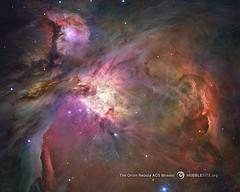### Thus SpeedIf we take R = 1megaparsek, the vap will be equal to 70.4 km / sec at the surface of a sphere of radius 1 megaparsek 1. Eslis surface photon is emitted in the direction of the center, its speed is equal to otnositelnoAP C-V (C-70, 4 km / sec) and this speed relative to the ap remains always ivezde. With this speed of photons arrive at the center of the sphere to the observer. In tsentresfery vap is zero, ie the observer is motionless relative to the ap eradicate the photon relative to the observer C-V (C-70, 4 km / sec). Thus The observer sees the photon, who flew to a distance of 1 Mpc, reddened, and decides that the light source is removed from it at a speed of 70.4 km / sec. It calculates the distance and was pleased that the Hubble constant is permissible limits (at plotnosti10-25kg/m3).

This is the Hubble constant. We have a choice: according to the accepted value of density we calculate the value of the Hubble constant, or measured the Hubble constant, we calculate the average density matter in the universe. As can be seen, the redshift and the Hubble znacheniepostoyannoy ap explains the properties without the involvement of "Big Bang" and "receding galaxies. According to the hypothesis up Big Bang was not, Vselennayaotnositelno stationary, respectively, did not need to invent an explanation of the fact that the Earth is older than her allotted Hubble age (14 billion years). be over 100 billion years.